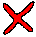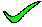The SQL produces the Cartesian product of the two tables. The number of elements in the Cartesian product is the number of rows of X multiplied by the number of rows of Y. The Cardinality is the number of rows.

closeThe SQL produces the Cartesian product of the two tables. The number of elements in the Cartesian product is the number of rows of X multiplied by the number of rows of Y. The Cardinality is the number of rows.

closeThe SQL produces the Cartesian product of the two tables. The number of elements in the Cartesian product is the number of rows of X multiplied by the number of rows of Y. The Cardinality is the number of rows.

closeThe SQL produces the Cartesian product of the two tables. The number of elements in the Cartesian product is the number of rows of X multiplied by the number of rows of Y. The Cardinality is the number of rows.

closeThe SQL produces the Cartesian product of the two tables. The number of elements in the Cartesian product is the number of rows of X multiplied by the number of rows of Y. The Cardinality is the number of rows.

close

 A B C D E TELL ME NEXT INDEX

There are two relations X and Y. Relation X has arity 1 and cardinality 2, relation Y has arity 3 and cardinality 4. Indicate the result of the SQL statement SELECT COUNT(*) FROM X,Y.

1. 4

2. 6

3. 8

4. Cannot be calculated from the information given

5. None of the above.